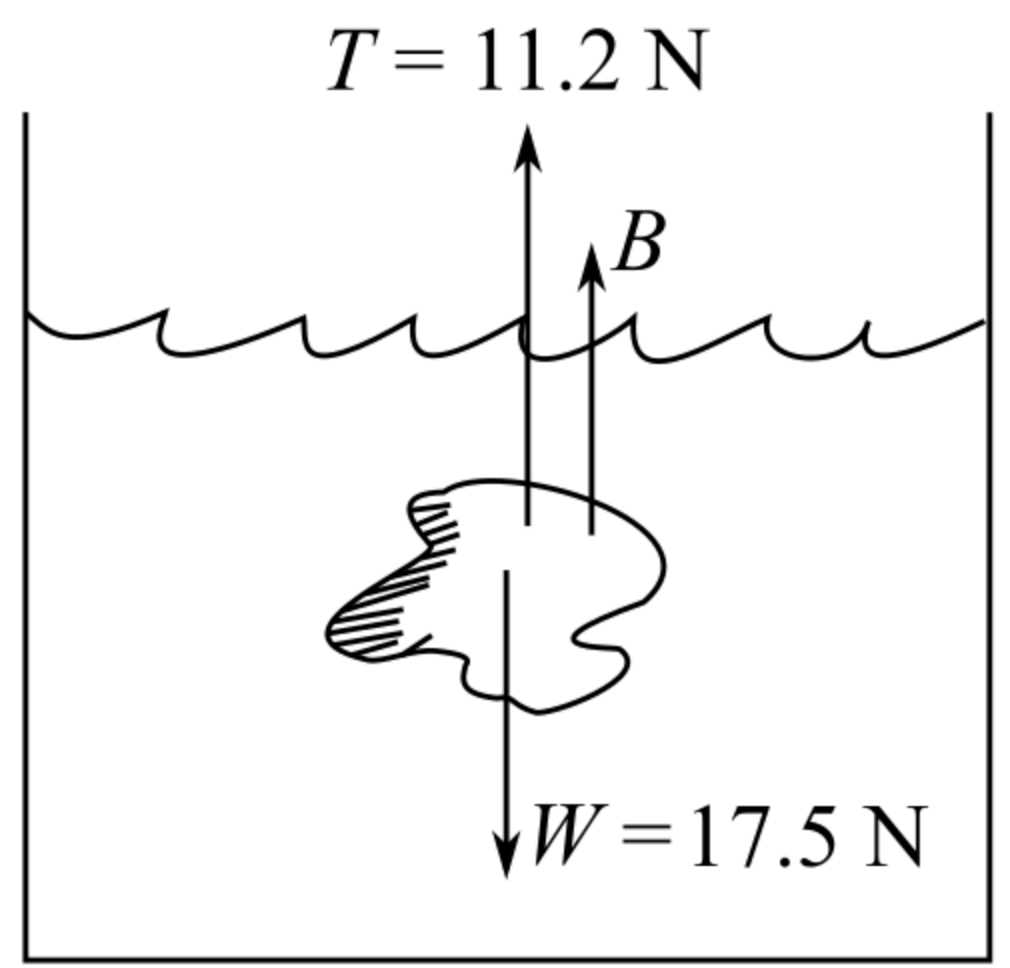In: Physics

# An ore sample weighs 17.50 N in air. when the sample is suspended

An ore sample weighs 17.50 N in air. when the sample is suspended by a light cord and totally immersed in water the tension in the cord is 11.20 N.

Find the total volume and density of the sample.

## Solutions

##### Expert Solution

Concepts and reason

This question is based on the concept of the equilibrium condition of forces, volume and density of the sample. Firstly, draw the diagram for sample. Calculate the buoyant force by using equilibrium condition of forces then calculate the volume of the sample and then calculate the density of the sample.

Fundamentals

The equilibrium condition of forces concurrent force can be written as, $$\sum F_{x}=0, \sum F_{y}=0$$ Here, $$\sum F_{x}$$ is the summation of horizontal force and $$\sum F_{y}$$ is the summation of vertical force. The expression for the buoyant force is, $$F_{b}=V g \rho_{w}$$

Here, $$V$$ is the volume of the sample, $$g$$ is the acceleration due to gravity and $$\rho_{w}$$ is the density of the water. The expression for density of sample is, $$\rho=\frac{m}{V}$$

Here, $$m$$ is the mass and $$V$$ is the volume of the sample. The expression for weight is, $$W=m g$$

Here, $$m$$ is the mass and $$g$$ is the acceleration due to gravity.

Diagram of sample is given as,Above diagram shows there are two forces applied in the cord. $$F_{b}$$ is the buoyant force and $$T$$ is the tension in the cord. Weight of the sample is towards the downward so use negative sign. Apply equilibrium condition of forces to find the buoyant force.

The equilibrium condition of forces concurrent force can be written as, $$\sum F_{x}=0, \sum F_{y}=0$$ From the diagram, $$T+F_{b}-W=0$$

Rearrange the equation for buoyant force. $$F_{b}=W-T$$

Substitute $$17.5 \mathrm{~N}$$ for $$W$$ and $$11.2 \mathrm{~N}$$ for $$T$$ in above expression.

$$\begin{array}{c} F_{b}=17.5 \mathrm{~N}-11.2 \mathrm{~N} \\ =6.3 \mathrm{~N} \end{array}$$

The expression for the buoyant force is, $$F_{b}=V g \rho_{w}$$

Rearrange the equation for volume of the sample. $$V=\frac{F b}{g o}$$

Substitute $$6.3 \mathrm{~N}$$ for $$F_{b}, 1000 \mathrm{~kg} \cdot \mathrm{m}^{-3}$$ for $$\rho_{w}$$ and $$9.8 \mathrm{~m} \cdot \mathrm{s}^{-2}$$ for $$g$$ in the above equation.

\begin{aligned} V &=\frac{6.3 \mathrm{~N}}{\left(9.8 \mathrm{~m} \cdot \mathrm{s}^{-2}\right)\left(1000 \mathrm{~kg} \cdot \mathrm{m}^{-3}\right)} \\ &=6.42 \times 10^{-4} \mathrm{~m}^{3} \end{aligned}

Volume of the sample is $$6.42 \times 10^{-4} \mathrm{~m}^{3}$$

Use equilibrium conditions of the force to calculate the buoyant force. Rearrange the equation of force in terms of volume of the sample. Substitute value of the acceleration and density of the water in equation of volume and find the volume of the sample.

The expression for weight is, $$W=m g$$

Substitute $$9.8 \mathrm{~m} \cdot \mathrm{s}^{-2}$$ for $$g$$ and $$17.5 \mathrm{~N}$$ for $$W$$ in the above equation.

\begin{aligned} 17.5 \mathrm{~N} &=m\left(9.8 \mathrm{~m} \cdot \mathrm{s}^{-2}\right) \\ m &=\frac{17.5 \mathrm{~N}}{9.8 \mathrm{~m} \cdot \mathrm{s}^{-2}} \\ &=1.78 \mathrm{~kg} \end{aligned}

The expression for density of sample is, $$\rho=\frac{m}{V}$$

Substitute $$1.78 \mathrm{~kg}$$ for $$m$$ and $$6.42 \times 10^{-4} \mathrm{~m}^{3}$$ for $$V$$ in the above equation.

$$\begin{array}{c} \rho=\frac{1.78 \mathrm{~kg}}{6.42 \times 10^{-4} \mathrm{~m}^{3}} \\ =2772.58 \mathrm{~kg} \cdot \mathrm{m}^{-3} \end{array}$$

Density of the sample is $$2772.58 \mathrm{~kg} \cdot \mathrm{m}^{-3}$$

Weight of the sample is product of mass of the sample and acceleration due to gravity. To calculate the mass of the sample use equation of weight. Substitute the value of mass and volume of sample in equation of density and calculate the density of the sample.

Volume of the sample is $$6.42 \times 10^{-4} \mathrm{~m}^{3}$$.

Density of the sample is $$2772.58 \mathrm{~kg} \cdot \mathrm{m}^{-3}$$.